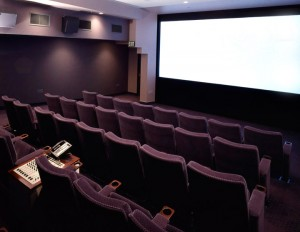# Photo NotesA place to talk about making images.

## July 10, 2009

### Understanding the Aperture

Filed under: Basic Photo Technique — John Siskin @ 1:14 pm

There is no other subject in teaching photography that is at the same time so basic and so important as understanding aperture and still so confusing and hard to explain. I want to start with a simple comparison to shutter speed: as the shutter speed number increases the amount of light let in by the shutter decreases. This is because the number is a fraction, and we are talking about the bottom number of the fraction, called the denominator. The number we use to discuss the aperture is also the bottom number of a fraction and as this number gets bigger the amount of light transmitted by the lens gets smaller. So for instance f16 lets less light reach the sensor than f8. Two stops less light, but we will get to that soon.

The aperture is a hole, which can be varied in size, in the middle of the lens. It blocks some of the light coming through the lens. The aperture number is actually the focal length of the lens divided by the width of the lens. So if you have a simple one-element lens that is one inch wide and has a focal length of 8 inches the lens aperture would be f8. If the lens had a one inch diameter and had a focal length of 4 inches that the aperture would be f4. Please don’t make me do this in metric, the relationships works but the math is more annoying. That wasn’t so bad was it? Here’s where it gets tricky, if you want the aperture to increase the light coming through the lens by one stop you have to double the area of hole in the middle of the lens. If you double the diameter (distance across the lens) you will increase the area of the circle to 4 times the originals size, or two stops. Instead of doubling the diameter of the lens you have to divide by the √2 (square root of two) which is approximately 1.4. This is similar to converting a focal length from your sensor size to what it would be in full frame 35mm. So 8÷1.4=5.714, which we refer to as f5.6. If you want to decrease the amount of light reaching the sensor by one stop you need to multiply by 1.4, remember the number must be smaller to let in more light. So 8 X 1.4=11.2, which we refer to as f11. It is important to remember that since we are working with fractions, things get turned around.

Please forgive me for all of this, but I didn’t make it up. As you may find it difficult to multiply by 1.4 in your head you may want some help on how to manipulate and use these numbers. There are a number of important applications that I will probably blog about later. In the mean time you might want to try to remember the full stops: 1, 1.4, 2, 2.8, 4, 5.6, 11, 16 and 22. You may notice that every other number is double; this helps to memorize the numbers.This shot uses long depth of field to keep the control panel and the room in focus

I also want to mention that small numbers isolate focus and large numbers increase the area. This is called depth of field. I wanted to mention it so that I can put pictures into this blog.
I am going to write about using the aperture to control the depth of field very soon, but I thought this was enough confusion for one week. People often tell me that they don’t need to know these things, and that is certainly true. But I want to control what happens in my pictures; I want to make pictures rather than just take pictures. If I understand how my camera records light I will, inevitably, have more control over my pictures.